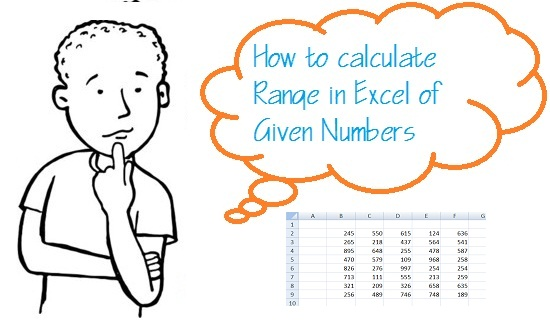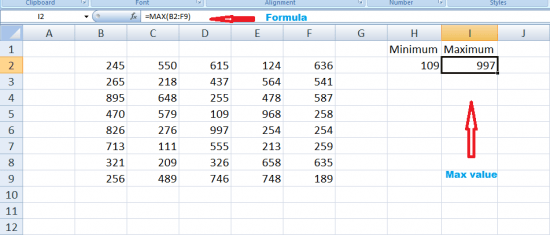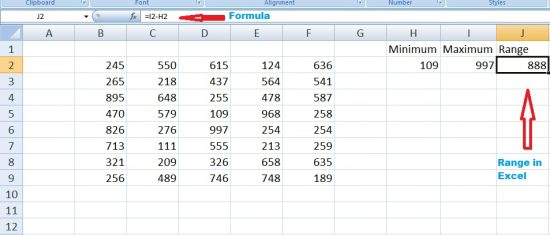# How to Calculate Range in Excel of Numbers using Excel Range Function

Microsoft Excel has very useful functions and we will discuss one of them, which will help to calculate range in excel for the given data set.

In order to find out the smallest and largest values in a range of numbers manually is typically not a handy work, especially when numbers are in contiguous form with large numbers of data. But thanks to excel range function, by using this formula we can calculate range in excel easily.

Not this excel range function is usually applicable to MS Excel 2007 and above versions.## How to Calculate Range In Excel for the given range of data set:

To calculate range in excel for given range of data, First, we will find the smallest and largest values in given range in excel using simple formula and then find out the difference in numbers using excel range formula. So follow the steps given below:

1 – Use the MIN function to find out the smallest value in the range of data. The formula of “=MIN(range)” is used to locate the smallest value, whereas “Range” means the range of cells.In the given example, =MIN(B2:F9) or the cells separated by commas, =MIN(B2,B6,C3,D4)

The Cell H2 Show the Minimum value in the given range of numbers in Excel.

2 – Use the MAX Function to find the largest value or number in the given data range. The formula “=MAX(range) “ is used, as an example you can replace values with the numbers used, e.g, =MAX(B2:F9)

The Cell I2 will show the Maximum value in the given range of numbers.3 – Subtract the Minimum Value from the Maximum Value using the format =Max-Min, as an example, we will use =I2-H2, where I2 shows the maximum and H2 shows the minimum value in the given range of numbers in Excel.In this way, you can easily calculate range in excel of given numbers using excel range function.

Do comments, if you have any query and don’t miss to read ” how to convert numbers into words in excel“.

Watch the video to learn how to calculate range in excel.